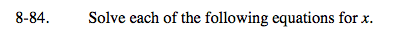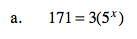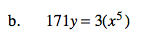Home > A2C > Chapter 8 > Lesson 8.1.5 > Problem8-84

8-84.
1. Solve each of the following equations for x. Homework Help ✎

1. 171 = 3(5x)

2. 171y = 3(x5)Simplify, then use logarithms.Use fractional exponents or roots.Your answer will be in terms of y.